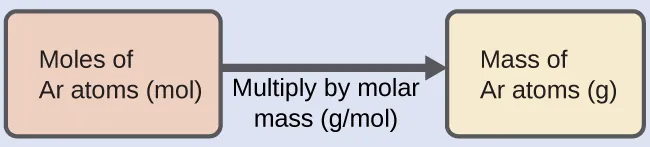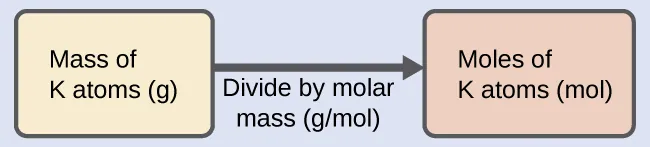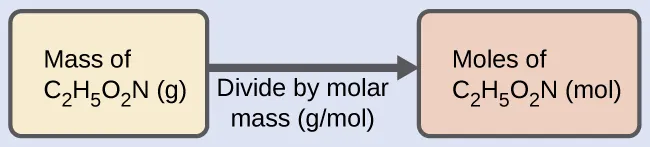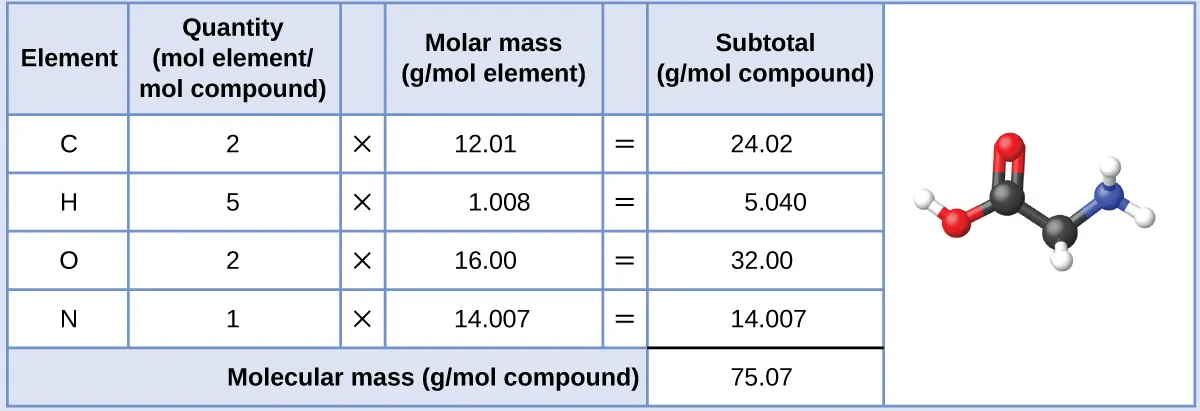# 17

### Moles to Mass

Where can you find the molar mass of the elements? The periodic table. You should always have one on hand—don’t expect to get very far without one!

How heavy is 1.5 mol of lead? How many moles in 22.34g of water? Calculating the mass of a sample from the number of moles it contains is quite simple. We use the molar mass (mass of one mole) of the substance to convert between mass and moles. When writing calculations, we denote the molar mass of a substance by an upper case “M” (e.g. M(Ne) means “the molar mass of neon”). As always, “n” stands for the number of moles and “m” indicates the mass of a substance. To find the solutions to the two questions we just asked, let’s apply some dimensional analysis:Can you see how the units cancel to give you the answer you want? All you needed to know was that you had 1.5 mol Pb (lead), and that 1 mol Pb weighs 207.2 grams. Thus, multiplying 1.5 mol Pb by 207.2 g Pb and dividing by 1 mol Pb gives you 310.8 g Pb, your answer.

Deriving Grams from Moles for an Element

A liter of air contains 9.2 × 10−4 mol argon. What is the mass of Ar in a liter of air?

Solution

The molar amount of Ar is provided and must be used to derive the corresponding mass in grams. Since the amount of Ar is less than 1 mole, the mass will be less than the mass of 1 mole of Ar, approximately 40 g. The molar amount in question is approximately one-one thousandth (~10−3) of a mole, and so the corresponding mass should be roughly one-one thousandth of the molar mass (~0.04 g):

###In this case, logic dictates (and the factor-label method supports) multiplying the provided amount (mol) by the molar mass (g/mol):

9.2×10−4 mol Ar (39.95 g/ mol Ar0.037g Ar

The result is in agreement with our expectations, around 0.04 g Ar.

What is the mass of 2.561 mol of gold?

504.4 g

Deriving Grams from Moles for a Compound

Vitamin C is a covalent compound with the molecular formula C6H8O6. The recommended daily dietary allowance of vitamin C for children aged 4–8 years is 1.42 × 10−4 mol. What is the mass of this allowance in grams?

Solution

As for elements, the mass of a compound can be derived from its molar amount as shown:The molar mass for this compound is computed to be 176.124 g/mol. The given number of moles is a very small fraction of a mole (~10−4 or one-ten thousandth); therefore, we would expect the corresponding mass to be about one-ten thousandth of the molar mass (~0.02 g). Performing the calculation, we get:

1.42×10−4 mol vitamin C (176.124 g/mol vitamin C0.0250g vitamin C

This is consistent with the anticipated result.

What is the mass of 0.443 mol of hydrazine, N2H4?

### Mass to Moles

But we had one more question: “How many moles in 22.34 g of water?” This is just as easy:Where did the 18 g H2O come from? We looked at the periodic table and simply added up the atomic masses of two hydrogens and an oxygen to get the molecular weight of water. This turned out to be 18, and since all the masses on the periodic table are given with respect to 1 mole, we knew that 1 mol of water weighed 18 grams. This gave us the relationship above, which is really just (again) watching units cancel out!

Deriving Moles from Grams for an Element

According to nutritional guidelines from the US Department of Agriculture, the estimated average requirement for dietary potassium is 4.7 g. What is the estimated average requirement of potassium in moles?

Solution

The mass of K is provided, and the corresponding amount of K in moles is requested. Referring to the periodic table, the atomic mass of K is 39.10 amu, and so its molar mass is 39.10 g/mol. The given mass of K (4.7 g) is a bit more than one-tenth the molar mass (39.10 g), so a reasonable “ballpark” estimate of the number of moles would be slightly greater than 0.1 mol.

The molar amount of a substance may be calculated by dividing its mass (g) by its molar mass (g/mol):

###The factor-label method supports this mathematical approach since the unit “g” cancels and the answer has units of “mol:”

4.7 (mol K / 39.10 g0.12 mol K

The calculated magnitude (0.12 mol K) is consistent with our ballpark expectation, since it is a bit greater than 0.1 mol.

Beryllium is a light metal used to fabricate transparent X-ray windows for medical imaging instruments. How many moles of Be are in a thin-foil window weighing 3.24 g?

Deriving Moles from Grams for a Compound

Our bodies synthesize protein from amino acids. One of these amino acids is glycine, which has the molecular formula C2H5O2N. How many moles of glycine molecules are contained in 28.35 g of glycine?

Solution

We can derive the number of moles of a compound from its mass following the same procedure we used for an element in Example 2.7:The molar mass of glycine is required for this calculation, and it is computed in the same fashion as its molecular mass. One mole of glycine, C2H5O2N, contains 2 moles of carbon, 5 moles of hydrogen, 2 moles of oxygen, and 1 mole of nitrogen:The provided mass of glycine (~28 g) is a bit more than one-third the molar mass (~75 g/mol), so we would expect the computed result to be a bit greater than one-third of a mole (~0.33 mol). Dividing the compound’s mass by its molar mass yields:

28.35 glycine (mol glycine / 75.07 g0.378 mol glycine

This result is consistent with our rough estimate.

How many moles of sucrose, C12H22O11, are in a 25-g sample of sucrose?

### Calculating Molar Masses

Before we can do these types of calculations, we first have to know the molar mass. Fortunately, this is not difficult, as the molar mass of an element is exactly the same as the atomic weight of that element. A table of atomic weights can be used to find the molar mass of elements (this information is often included in the periodic table). For example, the atomic weight of oxygen is 16.00 amu, so its molar mass is 16.00 g/mol.

For species with more than one element, we simply add up the atomic weights of each element to obtain the molar mass of the compound. For example, sulfur trioxide gas is made up of sulfur and oxygen, whose atomic weights are 32.06 and 16.00 respectively.The procedure for more complex compounds is essentially the same. Aluminium carbonate, for example, contains aluminium, carbon, and oxygen. To find the molar mass, we have to be careful to find the total number of atoms of each element. Three carbonate ions each containing three oxygen atoms gives a total of nine oxygens. The atomic weights of aluminium and carbon are 26.98 and 12.01 respectively.As described in the previous section, molar mass is expressed as “grams per mole”. The word per in this context implies a mathematical relationship between grams and mole. Think of this as a ratio. The fact that a per relationship, ratio, exists between grams and moles implies that you can use dimensional analysis to interconvert between the two. For example, if we wanted to know the mass of 0.50 mole of molecular hydrogen (H2) we could set up the following equations:

The known molar mass of H2 is:

2.03 H2/moH2

We are given that we have 0.50 moles of H2 and we want to find the number of grams of H2 that this represents. To perform the dimensional analysis, we arrange the known and the given so that the units cancel, leaving only the units of the item we want to find.EXERCISE

1. Determine the mass of 0.752 mol of H2 gas.
2. How many moles of molecular hydrogen are present in 6.022 grams of H2?
3. If you have 22.414 grams of Cl2, how many moles of molecular chlorine do you have?